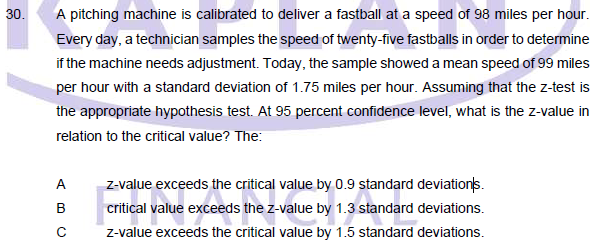# Question on Hypothesis Test, Z-valueHey guys, may I ask if this question the answer is B?

Z value = X-u / σ
= 98-99/ 1.75
= -0.57

So the Critical Value is 1.96 (Given that 95% confidence level) and the Z value is -0.57

1.96-0.57 = 1.39

Also may I ask how do I know this is a One tailed or Two tailed test?

I would go one tail test: pitchers pride themselves on their fastball, right @S2000magician???

Assuming a one tailed test, this implies 5% in the tail, which means a critical value of 1.645. As well, the null hypothesis is mean = 98, so the z-score is as follows:

X-u / (σ/5)
= (99-98)/ (1.75/5)
= 1/0.35
= 2.85

I will leave the rest to you.1 Like

I played Little League, Pony, and Colt ball till I was 16.

I never had a particularly hard fastball – high 80s, low 90s I’d imagine – but I had a curveball that would break at least 2 feet (65 cm). I could throw it at a right-hand batter’s head and it would break to the middle of the strike zone at his knees.

2 Likes